Sie sind hier: ICP » R. Hilfer » Publikationen

# IV.A Examples

The present chapter begins the discussion of physical processes in porous media involving the transport or relaxation of physical quantities such as energy, momentum, mass or charge. As discussed in the introduction physical properties require equations of motion describing the underlying physical processes. Recurrent examples of experimental, theoretical and practical importance include :

• the disordered diffusion equation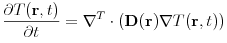(4.1)

where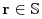,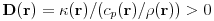is the thermal diffusivity tensor,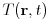is the space time dependent temperature field,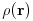is the density,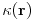the thermal conductivity and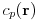the specific heat at constant pressure. The superscript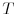denotes transposition. If the tensor field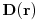is sufficiently often differentiable the equations are completed with boundary conditions at the sample boundary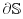. For the microscopic description of diffusion in a two component porous medium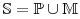whose components have diffusivities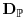and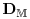the diffusivity fieldhas the form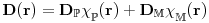which is not differentiable at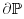. In such cases additional boundary conditions are required at the internal interface, and the equation is interpreted in the sense of distributions . Typical values for sedimentary rocks are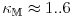Wm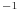K,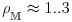g cm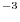and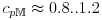kJ kgK.

• the Laplace equation with variable coefficients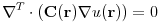(4.2)

where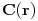is again a second rank tensor field of local transport coefficients,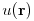is a scalar field,and the same remarks apply as for the diffusion equation with respect to differentiability ofand boundary conditions. For constantthe equation reduces to the Laplace equation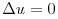. If the medium is random the coefficient matrix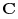is a random function of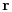. Equation (4.2) is the basic equation for the next chapter. It is frequently obtained as the steady state limit of the timedependent equations such as the diffusion equation (4.1). Other examples of (4.2) occur in fluid flow, dielectric relaxation, or dispersion in porous media. In dielectric relaxation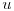is the electric potential andis the matrix of local (spatially varying) dielectric permittivity. In diffusion problems or heat flowis the concentration field or temperature, andthe local diffusivity. In Darcy flow through porous mediais the pressure andis the tensor of locally varying absolute permeabilities.

• the elastic wave equation is a system of equations for the three components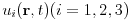of a vector displacement field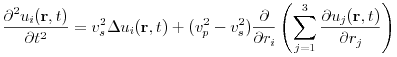(4.3)

where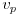is the compressional and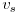the shear wave velocity of the material.

• Maxwells equations in SI units for a medium with real dielectric constant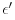, magnetic permeability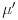and real conductivity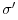and charge density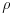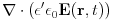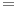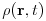(4.4)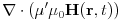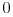(4.5)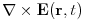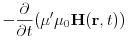(4.6)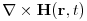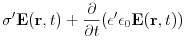(4.7)

for the electric field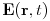, magnetic field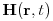supplemented by boundary conditions and the continuity equation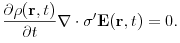(4.8)

Here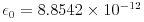F/m is the permittivity and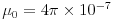H/m is the magnetic permeability of empty space.

• the Navier-Stokes equations for the velocity field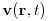and the pressure field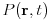of an incompressible liquid flowing through the pore space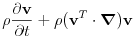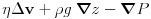(4.9)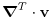(4.10)

whereis the density and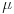the viscosity of the liquid. The coordinate system was chosen such that the acceleration of gravity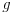points in the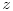-direction. These equations have to be supplemented with the no slip boundary condition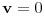on the pore space boundary.

In the following mainly the equations for fluid transport and Maxwells equation for dielectric relaxation will be discussed in more detail. Combining fluid flow and diffusion into convection-diffusion equations yields the standard description for solute and contaminant transport [260, 261, 24, 262, 21, 26].